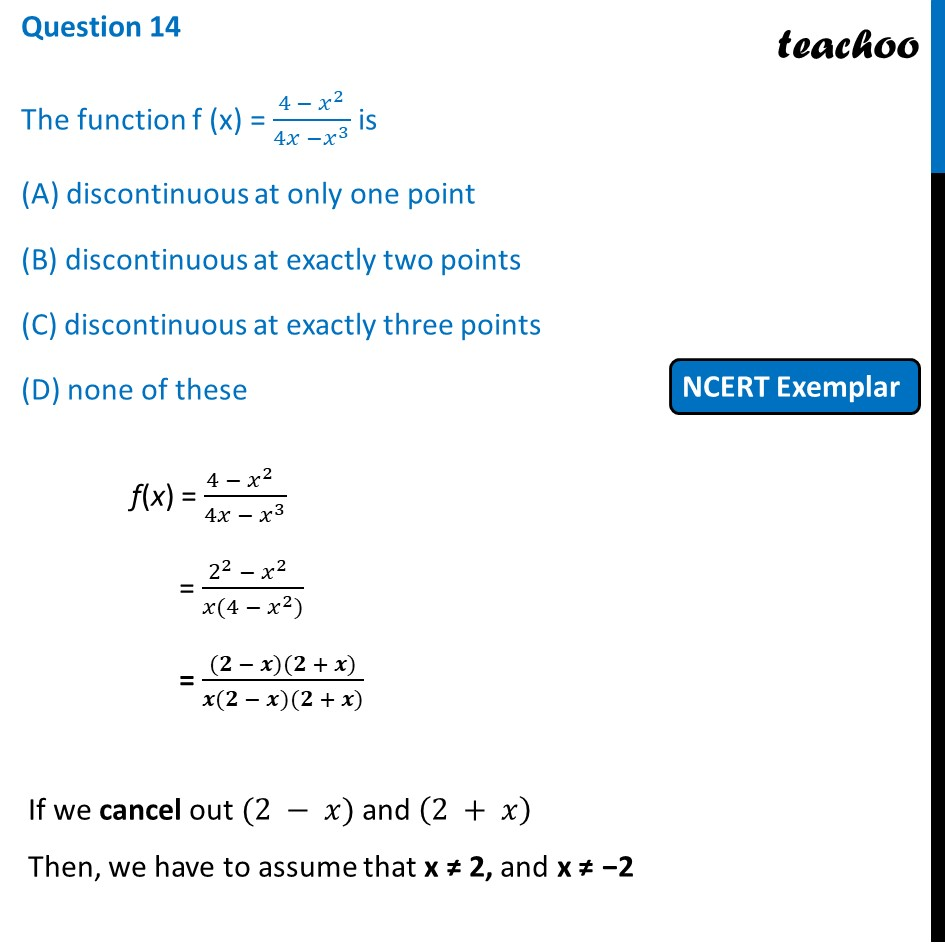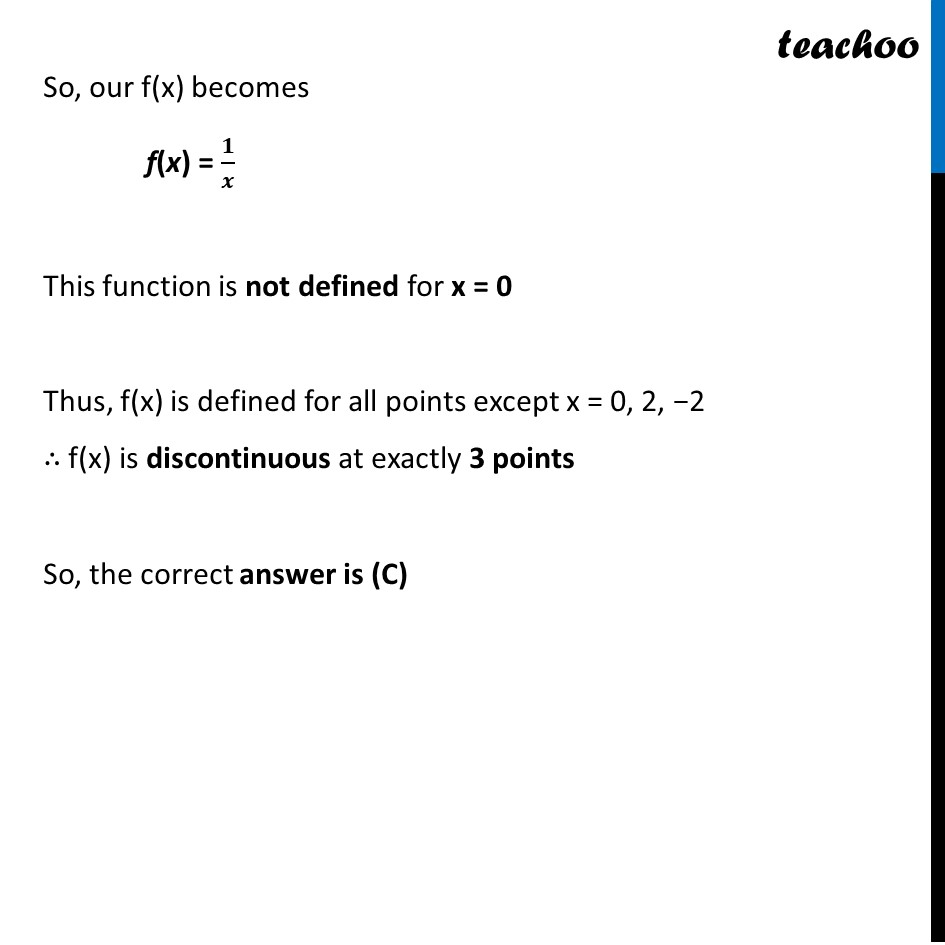NCERT Exemplar - MCQs

Chapter 5 Class 12 Continuity and Differentiability
Serial order wise

## (D) none of these

This question is similar to Ex 5.1, 3 (c) - Chapter 5 Class 12 - Continuity and DifferentiabilityLearn in your speed, with individual attention - Teachoo Maths 1-on-1 Class

### Transcript

Question 12 The function f (x) = (4 − 𝑥^2)/(4𝑥 −𝑥^3 ) is (A) discontinuous at only one point (B) discontinuous at exactly two points (C) discontinuous at exactly three points (D) none of these f(x) = (4 − 𝑥^(2 ) )/(4𝑥 − 𝑥^3 ) = (2^2 − 𝑥^(2 ) )/(𝑥(4 − 𝑥^2)) = ((𝟐 − 𝒙)(𝟐 + 𝒙))/(𝒙(𝟐 − 𝒙)(𝟐 + 𝒙)) If we cancel out (2 − 𝑥) and (2 + 𝑥) Then, we have to assume that x ≠ 2, and x ≠ −2 So, our f(x) becomes f(x) = 𝟏/𝒙 This function is not defined for x = 0 Thus, f(x) is defined for all points except x = 0, 2, −2 ∴ f(x) is discontinuous at exactly 3 points So, the correct answer is (C)